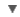WORDNET DICTIONARY
CIDE DICTIONARY
Link, Gadget and Share#parity bit

RELATED WORDS :

## WORDNET DICTIONARY

### Noun parity bit has 1 sense

• parity bit(n = noun.quantity) check bit, parity - (computer science) a bit that is used in an error detection procedure in which a 0 or 1 is added to each group of bits so that it will have either an odd number of 1's or an even number of 1's; e.g., if the parity is odd then any group of bits that arrives with an even number of 1's must contain an error;
• is a kind of bit

## CIDE DICTIONARY

parity bitn.
The bit within a data structure which is assigned a value of 1 or 0 so as to make the parity{7} of the data structure odd or even. Data structures may or may not have parity bits, dpending on whether the system does or does not perform parity checking. The most commonly used parity bit is the eigth (higher-order) bit of a byte, which is used when data transmission uses only the 7 lower-order bits of each byte as significant data; some memory systems use a ninth bit as a parity bit for each eight bits (one byte) of significant data in memory.  [PJC]
copyright © 2012 Yayasan Lembaga SABDA (YLSA) | To report a problem/suggestion